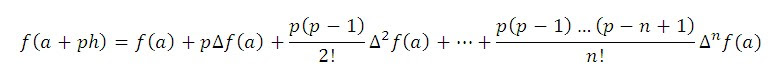# Newton's Forward Interpolation Formula with MATLAB Program

In everyday life, sometimes we may require finding some unknown value with the given set of observations. For example, the data available for the premium, payable for a policy of Rs.1000 at age x, is for every fifth year. Suppose, the data given is for the ages 30, 35, 40, 45, 50 and we are required to find the value of the premium at the age of 42 years, which is not directly given in the table. Here we use the method of estimating an unknown value within the range with the help of given set of observation which is known as interpolation.

## Definition of Interpolation

Given the set of tabular values (x0, y0), (x1, y1),…,(xn, yn) satisfying the relation y=f(x) where the explicit nature of f(x) is not known, it is required to find a simpler function say ?(x), such that f(x) and ?(x) agree at the set of tabulated points. Such a process is called as interpolation.

If we know ‘n’ values of a function, we can get a polynomial of degree (n-1) whose graph passes through the corresponding points. Such a polynomial is used to estimate the values of the function at the values of x.

We will study two different interpolation formula based on finite differences when the values of x are equally spaced. The first formula is:

Newton’s forward difference interpolation formula:

The formula is stated as:Where ‘a+ph’ is the value for which the value of the function f(x) is to be estimated. Here ‘a’ is the initial value of x and ‘h’ is the interval of differencing.

## Question

The table gives the distance between nautical miles of the visible horizon for the given height in feet above the earth surface.  Find the value of y when x= 218 feet.

## MATLAB Program for Newtons Forward Interpolation Formula

### You may also like

1.